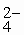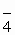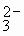CHEMISTRY-2004

 Time: 1 Hour Max.Marks : 60

Instructions:

(1) Answer must be written either in English or the medium of instruction of the candidate in high school.
(2) There will be no negative marking
(3) Use of calculators or graph papers is not permitted
(4) There are TWENTY FIVE (25) questions. Answer all the question.
(5) Questions 1-15 carries 2 marks each and questions 16-25 carries 3 marks each.

1. What is chemistry and what is the scope of this science?

2. What is the need to have different theories of acids and bases?

3. What is the molality and molarity of pure ethyl alcohol at 250C, the density of ethyl alcohol is 0.92 g/cc?

4. What is the authentic test for detection of nitrate ion in the laboratory? Explain with proper equations.

5. Temporary hardness of water can be removed by heating. Explain?

6. Define the terms strong and weak electrolytes. How are they differentiated?

7. Why cannot all minerals of a metal be used for the extraction of metal?

8. What is the rate constant of reaction and how it is related to equilibrium constant?

9. What is the meaning of ionic product of water? Why and how does it depend on temperature?

10. What is a deliquescent substance and under what conditions does a hydrated salt show deliquescent property.

11. BF3 acts as a Lewis acid whereas NF3 does not. Explain?

12. Sodium has higher density than Lithium, but potassium has lower density than sodium. Why?

13. NH4+ has a bond angle nearly equal to bond angle of CH4 but ammonia has a different bond angle. Why?

14. How does elemental nitrogen present in the atmosphere is enter into soil in the form of nitrate?

15. Write the cathodic and anodic reactions when aqueous solution of Na2SO4 is electrolysed with platinum electrodes.

16. Why is strength of Hydrogen peroxide is expressed in volumes?. Calculate the strength of 5 volumes of hydrogen peroxide in molarity?

17. Rate of reaction depends on concentration of the reactants. At equilibrium rate of forward and backward reactions is same but concentration of reactants and products is not same. Explain?

18. Equilibria of all chemical reactions are not affected by change of pressure. Explain?

19. Write the principle involved in the froth floatation process?

20. Sodium chloride and sodium iodide on reaction with H3PO4 produce HCl and HI respectively, but sodium chloride produces HCl whereas sodium iodide produces iodine when treated with H2SO4. Explain?

21. A purple solution of aqueous potassium permanganate (KMnO4) reacts with aqueous sodium sulphite (Na2SO3) in the basic solution to yield the green manganate ion (MnO) and sulphate ion (SO). The unbalanced equation is
MnO(aq) + SO(aq) ® MnO(aq)+ SO(aq).

a. Identify the oxidant and reductant
b. Balance the chemical equation by oxidation number method

22. Aluminium cannot be extracted from Al2O3 by carbon reduction method. Why? And write the principle involved in the extraction of aluminium from Al2O3 through chemical equations.

23. Aluminium and Zinc hydroxides are insoluble in water but both are soluble in NaOH even though NaOH contains common ion. Why?

24. Why does nitrogen form stable diatomic N2 whereas phosphorus gives P4?

25. Heat of combustion of acetylene is less than that of ethane and ethylene but oxyacetylene flame is used for welding and cutting of metals. Why?